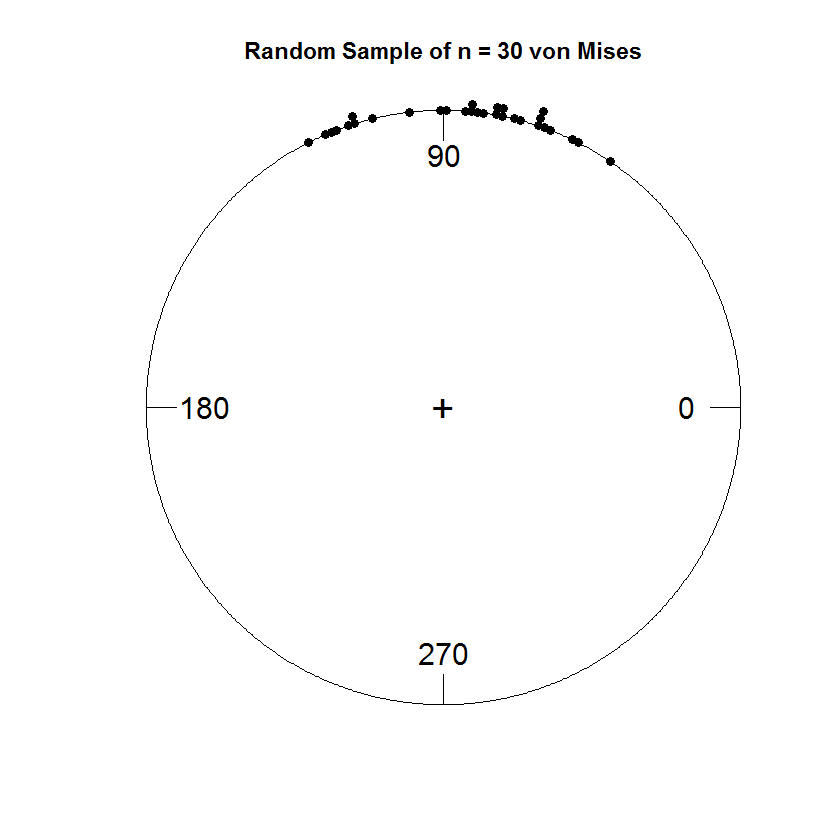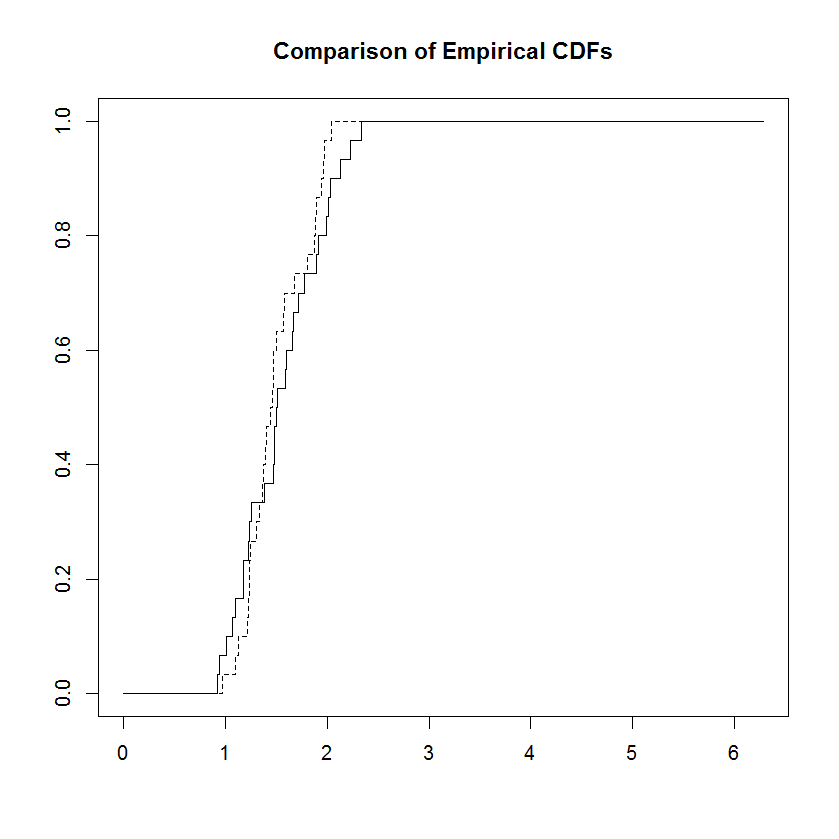Data Science Design Pattern #4: Transformations of Individual VariablesData Science Design Pattern #4:Transformations of Individual Variables

posted by Mosaic Data Science

It’s very common while exploring data to experiment with transformations of individual variables.  Some transformations rescale while preserving order; others change both scale and order.  In this post we describe some common ways to transform individual variables, and explore how doing so may benefit an analysis.  (We’ll tackle transformations of multiple variables in another post.)

Description

Rescaling while preserving order.  Sometimes you need to re-scale a source variable without changing the order relations among its possible values.  This can reveal a pattern or let us use a more tractable distribution or computational technique.[i]  Transformations that preserve order are termed positive monotonic.[ii]

The set of positive monotonic transformations is large.  In most cases the transformation you want becomes obvious, once you’re clear what properties you want the transformed variable to have.  In fact, sometimes it’s easier to work backwards from the transformed variable you want to your source variable, to identify the appropriate transformation.

The most frequently used order-preserving transformation in statistics is standardizing, which means subtracting the population mean (centering) and then dividing by the population standard deviation (rescaling).  Standardizing produces a dimensionless quantity:  regardless of the units you start out with, you end up with the same values.  Standardizing turns a variable into a standardized variable or Z-score.[iii]  When you use the sample mean and standard deviation, you end up with a t-statistic, which has similar (but not necessarily identical) statistical properties.[iv]

Another common rescaling transformation is the logarithm.  Logarithms can turn exponentials into linear functions, making them easier to handle.  Logarithms can also center proportions and make them symmetrical.  For example, you may wish to study a proportion between two positive variables X and Y.  One approach would be to express each variable as a fraction of the two variables’ sum:  X/(X+Y).  You could also consider the fraction X/Y.  The problem in both cases involves scaling and centering.  If you reverse the roles of X and Y, you get different magnitudes.  For example, if X = 1 and Y = 10, X/(X+Y) = 1/11 while Y/(X+Y) = 10/11 (and the central value when X = Y is one-half, not zero).  Likewise X/Y = 1/10 while Y/X is 10/1 (and the central value is again non-zero).  But log10(X/Y) has symmetric magnitudes that are opposite in sign, and the function is symmetrical around zero.  Thus if X = 1 and Y = 10, log10(X/Y) = -1, while if X = 10 and Y = 1, log10(X/Y) = 1.

Order-changing transformations.  Sometimes a source variable’s nominal ordering masks underlying natural adjacency relationships.  Continuing our previous example (the log ratio), the case where X = 1 and Y = 10 is as far from the center as the case where X = 10 and Y = 1.  The log ratio gives these cases opposite signs.  If only the size (not the sign) of the deviation is of interest, you can take the absolute value:  |log10(X/Y)|.  Or, if you want also to penalize large deviations while ignoring sign, square the log ratio:  (log10(X/Y))2.  In either case the transformation changes the ordering of the proportion, as well as re-scaling it, while preserving or accentuating a property of interest (relative magnitude).

An important class of order-changing transformation treats cyclical data.  One common case is cyclical time variables such as the minute of the day or day of the year.  In both cases the nominal variable’s range is [1, n] for some positive integer n.  But the distance d(j, k) for 1 ≤ j ≤ k ≤ n (without loss of generality) is not simply k – j.  Rather, d(j, k) = min(k – j, n – k + j).  For example, in a 365-day year, d(14, 15) = 15 – 14 = 1.  But d(5, 360) = d(360, 365) + d(365, 1) + d(1, 5) = (365 – 360) + 1 + 4 = 10.  An equally important case is angles, especially latitudes and longitudes in geospatial data.  For example, d((180, 355), (180, 5)) = 10.

When what matters to us about time is its progression, we can avoid cyclical time variables by numbering units of time (e.g. days) sequentially, starting with a given epoch.  But where seasonality matters, we need a transformation that honors seasonal cycles.  Angular data likewise require a transformation that honors the circle or globe’s topology.  The field called circular statistics[v] provides these techniques.[vi]  The remainder of this post focuses on circular statistics.

How it Works

Transforming linear values to angles.  Circular variables have no natural zero or magnitude.  Thus converting a linear value (such as time of day) into an angular value implicitly converts the linear value into an angle from the circular origin.  For example, 6 a.m. is 1/4th of a day, so as an angular value it becomes 360 / 4 = 90.

Circular summary statistics.  Computing summary statistics is more complex on the circle.  For example, the mean θm of n angles {θ1, . . . , θn} is now the arctangent of the ratio of the mean sine and the mean cosine.  In symbols:

θm = arctan((∑1≤i≤n sin(θi)) / (∑1≤i≤n cos(θi)))

where the arctangent is interpreted to produce a positive angle.  It quickly becomes more natural to translate angles into complex unit vectors using Euler’s formula z = e = cos(θ) + i sin(θ), and to use the complex representation to compute central values, measures of dispersion, moments, etc.  For example, the sample mean vector, called the sample resultant, is rs = (∑1≤i≤n zi) / n, and θm = arg(rs), which has sample resultant length |rs| in [0, 1].  (Zero means uniform dispersion; one means all data points are at the same angle.)  The sample circular variance is 1 – |rs|.  For technical reasons the sample circular standard deviation is (-2ln(|r|))1/2, rather than the square root of the circular variance.[vii]

Distributions.  There are several common “wrapped” (circular) distributions.  The circular uniform distribution has density 1 / 2π, undefined mean, and resultant zero (hence unity circular variance).  The von Mises distribution (sometimes called the circular normal distribution) closely approximates the wrapped normal distribution.  The von Mises has two limiting distributions:  the circular uniform and the wrapped normal.  Its popularity lies in its analytical simplicity and its close approximation to the wrapped normal.  There are also wrapped Cauchy and Levy distributions.

Measures of dispersion.  There are several techniques for assessing dispersion in a circular data set.  For example, the Rayleigh Z test determines whether the sample data is oriented in some unspecified common direction (has a unimodal distribution with unknown mean and resultant length), or is randomly oriented.  The test computes a test statistic Z = nr2 and compares it to a critical value.[viii]  Another version of the Rayleigh test (the V test) tests an alternative hypothesis that the data have a unimodal distribution in a specified direction (still with unknown resultant length).[ix]  Rayleigh’s tests assume the data are unimodal, not diametrically bidirectional (bimodal, with clusters oriented at 180 to each other).  If the data are diametrically bidirectional, one can transform the data using the angle-doubling procedure.[x]

Goodness of fit.  There are goodness-of-fit tests for circular distributions.  For example, Watson’s test checks a data set for goodness of fit for circular uniform and von Mises distributions.[xi]

When to Use it

Use positive monotonic transformations to reshape a distribution in a way that underscores a pattern or makes computations more tractable.  Use order-changing transformations when you want to abstract away from order (at least partially), perhaps to focus on magnitude or direction.  Use circular statistics when seasonality or direction is the central concept.

Example

The R code in Figure 1 generates two random samples, each with n = 30.  The first is from a wrapped normal distribution; the second, a von Mises distribution.  Figure 2 plots the wrapped normal, and Figure 3 plots the von Mises.  The R code then uses Watson’s two-sample test of homogeneity to test the null hypothesis that the two samples are from the same distribution.  The test statistic’s value is 0.1323.  The critical value is 0.268.  Since the test statistic is less than the critical value, the null hypothesis cannot be rejected (at the p = 0.01 significance level).

set.seed(124)
# Generate and plot a random sample of 30 wrapped normal values.
wnSample <- sort(
rwrpnorm(
n = 30,
mu = pi * 0.5,
rho = 0.9
)
)
circ.plot(
x = wnSample,
main = “Random Sample of n = 30 Wrapped Normals”,
stack = TRUE,
bins = 300
)
# Generate and plot a random sample of 30 von Mises values.
vmSample <- sort(
rvm(
n = 30,
mean = pi * 0.5,
k = 8
)
)
circ.plot(
x = vmSample,
main = “Random Sample of n = 30 von Mises”,
stack = TRUE,
bins = 300
)
# Test whether the two samples come from the same population.
watson.two(
x = wnSample,
y = vmSample,
alpha = 0.01,
plot = TRUE
)

Figure 1:  R Code for Example Watson Two-Sample TestFigure 2:  Random Wrapped Normal SampleFigure 3:  Random von Mises SampleFigure 4:  Watson Two-Sample Test Plot Comparing Empirical CDFs

[i] Kernel methods are an extremely powerful family of techniques based on transformations into a feature space where inner products can be computed from the untransformed variables.  See John Shawe-Taylor & Nello Cristianini, Kernel Methods for Pattern Analysis (Cambridge University Press, 2009).  We will treat kernel methods in a separate post.

[v] The most recent comprehensive treatment is Arthur Pewsey and Markus Neuhauser, Circular Statistics in R (Oxford University Press, 2013).  See also N. I. Fisher, Statistical Analysis of Circular Data (Cambridge University Press, 1995).

[vi] The alternative our example suggests is to use a more complicated distance function.  This works in some cases.  But when e.g. summary statistics are computed, the modified-distance-function approach fails.  For example, the mean of 3 and 357 could be either 180 (the arithmetic mean) or 0 (the midpoint).  But rotate the axes by -6 to change the values to 351 and 357, and only one mean value (the midpoint 354) is possible—even though the angle between the two values has not changed.

[vii] Where the circular variable has a wrapped normal or von Mises distribution, the circular standard deviation estimates the standard deviation of the underlying linear normal distribution.  So you can use it to standardize circular values.  http://en.wikipedia.org/wiki/Directional_statistics#Measures_of_location_and_spread (visited March 10, 2014).

[viii] The r.test function in the R package CircStats implements the Rayleigh Z test.  See http://cran.at.r-project.org/web/packages/CircStats/CircStats.pdf (visited march 10, 2014).

[ix] The v0.test function in the R package CircStats implements the Rayleigh V test.  See http://cran.at.r-project.org/web/packages/CircStats/CircStats.pdf (visited March 10, 2014).  For examples of the Z and V tests, see http://facstaff.unca.edu/tforrest/BIOL%20360%20Animal%20Behavior/2012/Behavior%20Lab%20Circular%20Statistics.pdf (visited March 10, 2014).

[xi] The Watson function in the R package CircStats implements the Watson goodness of fit test.  See http://cran.at.r-project.org/web/packages/CircStats/CircStats.pdf (visited march 10, 2014).  The same package’s circ.range, kuiper, and rao.spacing functions can also test for uniformity.

This site uses Akismet to reduce spam. Learn how your comment data is processed.